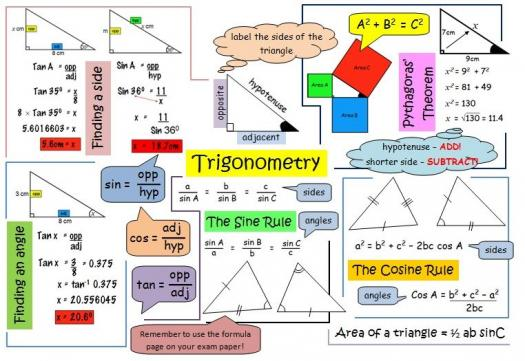# Trigonometry With General Triangles Assessment Test

Approved & Edited by ProProfs Editorial Team
At ProProfs Quizzes, our dedicated in-house team of experts takes pride in their work. With a sharp eye for detail, they meticulously review each quiz. This ensures that every quiz, taken by over 100 million users, meets our standards of accuracy, clarity, and engagement.
| Written by Cripstwick
C
Cripstwick
Community Contributor
Quizzes Created: 693 | Total Attempts: 678,987
Questions: 10 | Attempts: 216SettingsTake this assessment test to evaluate your knowledge of trigonometry with general triangles. What are sine, cosine, and tangent? How can we use them to solve for unknown sides and angles in general triangles?

• 1.

### What can be used to find all the missing angles of a triangle?

• A.

Graph

• B.

Protractor

• C.

Law of cosine

• D.

Compass

C. Law of cosine
Explanation
The Law of Cosines is a mathematical formula that can be used to find missing angles in a triangle. It relates the lengths of the sides of a triangle to the cosine of one of its angles. By knowing the lengths of two sides and the included angle, or the lengths of all three sides, the Law of Cosines can be used to calculate the missing angles. This makes it a useful tool for solving triangle problems involving missing angles.

Rate this question:

• 2.

### When two sides and an angle opposite one of them are known, it is called...

• A.

Ambiguous case

• B.

Retard Case

• C.

Right angle

• D.

Trapezium

A. Ambiguous case
Explanation
When two sides and an angle opposite one of them are known, it is called the ambiguous case. This means that there can be more than one possible solution for the triangle. In this case, additional information is needed to determine a unique triangle.

Rate this question:

• 3.

### Which side is the longest side of the right angle triangle?

• A.

• B.

Angle

• C.

Opposite

• D.

Hypotenuse

D. Hypotenuse
Explanation
The hypotenuse is the longest side of a right angle triangle. It is the side opposite the right angle and is always the longest side in a right triangle.

Rate this question:

• 4.

### What can be used to test whether a triangle has a right angle?

• A.

Pythagoras theorem

• B.

Set theory

• C.

Square combination

• D.

Compass rule

A. Pythagoras theorem
Explanation
The Pythagorean theorem can be used to test whether a triangle has a right angle. According to the theorem, in a right-angled triangle, the square of the length of the hypotenuse (the side opposite the right angle) is equal to the sum of the squares of the other two sides. By applying this theorem, one can determine if a triangle has a right angle by checking if the lengths of the sides satisfy this relationship.

Rate this question:

• 5.

### The unequal side of an isosceles triangle is referred to as...

• A.

Base

• B.

Hypotenuse

• C.

Angle

• D.

Line

A. Base
Explanation
In an isosceles triangle, two sides are equal in length, while the third side, which is different in length, is called the base. Therefore, the correct answer is "Base".

Rate this question:

• 6.

### When the 3rd angle of an isosceles triangle is a right angle, it is called...

• A.

Trapezium

• B.

Angular

• C.

Real angle

• D.

Right isosceles triangle

D. Right isosceles triangle
Explanation
When the 3rd angle of an isosceles triangle is a right angle, it is called a right isosceles triangle because it has two equal sides and one right angle. In a right isosceles triangle, the two equal sides are the legs, and the right angle is formed by the intersection of the legs. This type of triangle is special because it combines the properties of both a right triangle and an isosceles triangle.

Rate this question:

• 7.

### A perpendicular distance from the base of an isosceles triangle to the topmost vertex is called...

• A.

Altitude

• B.

Width

• C.

• D.

Height

A. Altitude
Explanation
The perpendicular distance from the base of an isosceles triangle to the topmost vertex is called the altitude.

Rate this question:

• 8.

### What is the area of a triangle?

• A.

BH

• B.

2bh

• C.

1/2bh

• D.

BBB

C. 1/2bh
Explanation
The correct answer is 1/2bh. The formula for finding the area of a triangle is 1/2 times the base (b) times the height (h). This formula is derived from the fact that a triangle can be divided into two equal right triangles, and the area of a right triangle is 1/2 times the base times the height. Therefore, to find the area of any triangle, we can use the formula 1/2bh.

Rate this question:

• 9.

### The sum of any 2 sides of a triangle must be greater than the measure of the third side is a postulate of...

• A.

Triangle Inequality Theorem

• B.

Pythagorean theorem

• C.

Triangle theorem

• D.

Line Law

A. Triangle Inequality Theorem
Explanation
The Triangle Inequality Theorem states that the sum of any two sides of a triangle must be greater than the measure of the third side. This is a fundamental postulate in geometry that helps determine if a given set of side lengths can form a valid triangle. It is used to ensure that the triangle's sides do not overlap or form a straight line, which would not create a triangle. Therefore, the correct answer is Triangle Inequality Theorem.

Rate this question:

• 10.

### What can be used to find the length of a side or size of an angle?

• A.

Cosine rule

• B.

Since rule

• C.

Tan rule

• D.

Angles ruleBack to top